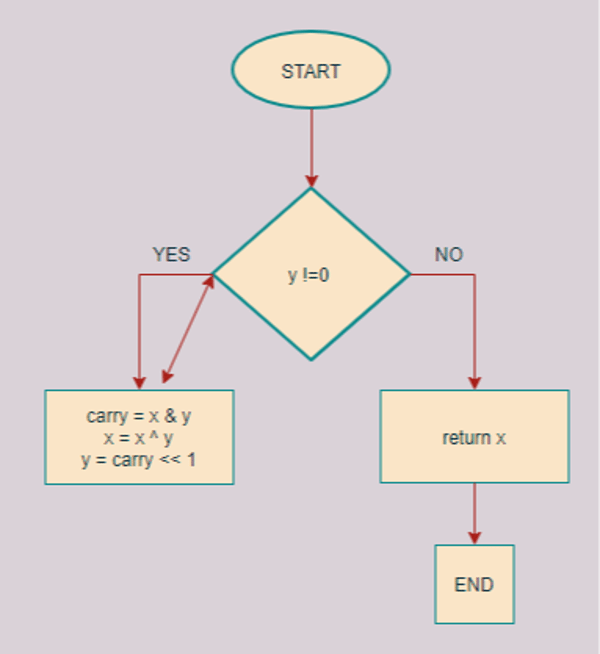Related Tags

communitycreator
python
bitwise

# How to add two integers without the addition operator in Python

Vinisha Maheshwari

Typically, the addition operator (+) is used to add two numbers, but to improve the time complexity of the operation, bitwise operators are a suitable alternative.

### Description

The sum of two numbers can be obtained by performing the XOR (^) operation on these numbers and adding a carry bit that is obtained by performing the AND (&) operation.

For example, if x and y are the numbers to be added, you can calculate (x & y) << 1 and add the result to x ^ y to get the desired sum. This concept is known as the half adder.

The flowchart below demonstrates the same example.Flow chart

### Code

The code to find the sum of two numbers without the addition operator in Python is shown below.

def Add(x, y):
while (y != 0):
carry = x & y
x = x ^ y
y = carry << 1
return x
print(Add(27,5))
Adding two numbers without using Arithmetic operators

### Explanation

The code above performs the following actions:

• In line 2, a while loop iterates until there is no carry bit left.

• In line 3, the carry bit is obtained through the bitwise AND (&) of the given numbers.

• Line 4 calculates the sum through the bitwise XOR (^) of the two numbers.

• In line 5, the code shifts the carry variable by one so that adding it to x gives the required sum.

RELATED TAGS

communitycreator
python
bitwise

CONTRIBUTOR

Vinisha Maheshwari
RELATED COURSES

View all Courses

Keep Exploring

Learn in-demand tech skills in half the time